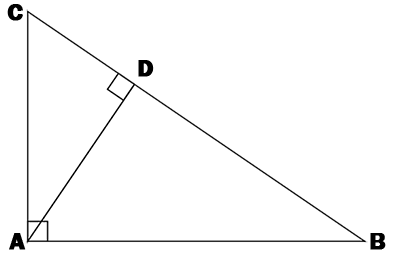### A right triangle ABC has the cathetus AB = 3 cm and the hypotenuse BC = 5 cm. Calculate the length of the AC cathetus.Fill in herecm

### Remember !

Pythagorean theorem is a relation between the three sides of a right triangle. The three sides are two cathetus and a hypotenuse.Pythagorean theorem definition. In any right triangle, the sum of the squares of the lengths of the cathetus is equal to the square of the length of the hypotenuse.

If we note with c - the hypotenuse and a, b the cathetus. Then the Pythagorean theorem can be written in the following form c² = a² + b².### Exemple !

Abbreviations: A - area, AB - cathetus, AC - cathetus, BC - hypotenuse and AD - height.1. A right triangle ABC has the cathetus AB = 3 cm and the hypotenuse BC = 5 cm. Calculate the length of the AC cathetus.

BC2 = AB2 + AC2

AC2 = BC2 - AB2

AC2 = 25 - 9

AC = √16

AC = 4 cm

2. A right triangle ABC has the cathetus AB = 3 cm and AC = 4 cm. Calculate the length of the hypotenuse BC.

BC2 = AB2 + AC2

BC2 = 9 + 16

BC = √25

BC = 5 cm

Site-ul nostru poate rămâne gratuit numai prin afișarea de reclame.

Vă rugăm să ne susțineți prin dezactivarea blocării de anunțuri.